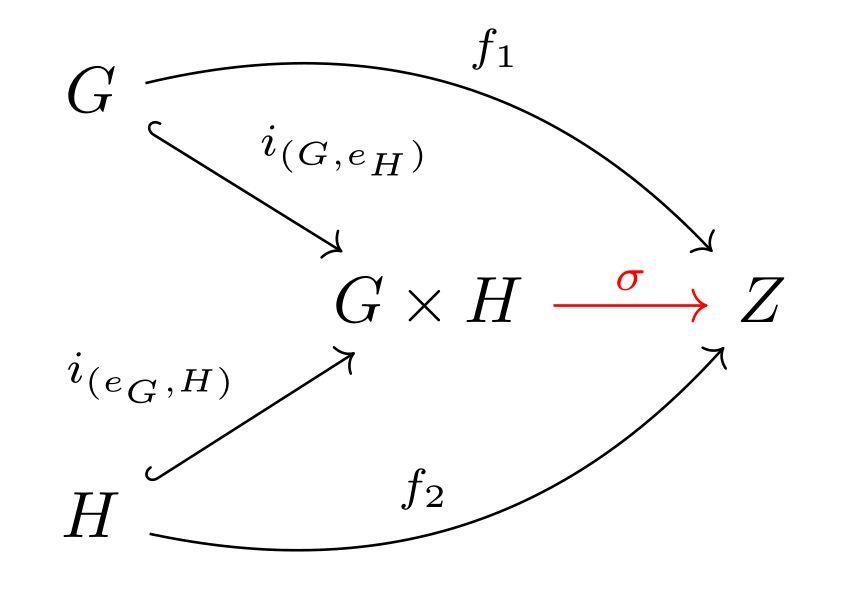Math and science::Algebra::Aluffi

# The set products satisfy the coproduct property in the Abelian category.

The set product, where elements are pairs, is capable of acting as a co-product object in the category $$\cat{Ab}$$. In other words, the following diagram commutes in $$\cat{Ab}$$ where $$\sigma$$ is uniquely determined by the rest of the diagram.

[:can you remember the commutative diagram?]### $$i_{\small{(G, e_H)}}$$ and $$i_{\small{(e_G, H)}}$$, the inclusion functions

The function $$i_{(G, e_H)} : G \to G \times H$$ and $$i_{(e_G, H)} : H \to G \times H$$ are special inclusion functions given by:

[\begin{align} i_{(G, e_H)}(a) &= \quad ? \\ i_{(e_G, H)}(i) &= \quad ? \end{align} ]

They place the input in either the first or second entry of a pair, leaving the other entry to be the identity element of the corresponding group, $$G$$ or $$H$$. The fixed identity entry allows the variable entry to inherit the group behaviour of the input. This insures that the inclusion functions satisfy the requirements to be [what?].

### The morphism $$\sigma$$

It is not a matter of searching for $$\sigma$$, for it's form was anticipated from the beginning.

$$\sigma : G \times H \to Z$$ can be expressed precisely in terms of $$f_1, f_2$$ and $$\groupMul{Z}$$:

[$\sigma(x, y) = \quad ?$ ]

The definition of $$\sigma$$ follows (is forced from) from the constraints. What is interesting is that $$\sigma$$ is actually possible! And only just. Can you remember why $$\sigma$$ works in $$\cat{Ab}$$ but not in $$\cat{Grp}$$?